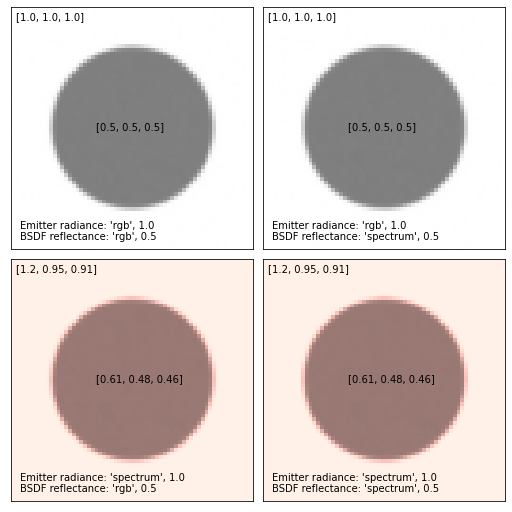# Spectra#

This section describes the plugins behind spectral reflectance or emission used in 3. On an implementation level, these behave very similarly to the texture plugins described earlier (but lacking their spatially varying property) and can thus be used similarly as either BSDF or emitter parameters:

<scene version=3.0.0>
<bsdf type=".. BSDF type ..">
<!-- Explicitly add a uniform spectrum plugin -->
<spectrum type=".. spectrum type .." name=".. parameter name ..">
<!-- Spectrum parameters go here -->
</spectrum>
</bsdf>
</scene>


In practice, it is however discouraged to instantiate plugins in this explicit way and the XML scene description parser directly parses a number of common (shorter) <spectrum> and <rgb> tags See the corresponding section about the scene file format for details.

The following two tables summarize which underlying plugins get instantiated in each case, accounting for differences between reflectance and emission properties and all different color modes. Each plugin is briefly summarized below.

XML description

Monochrome mode

RGB mode

Spectral mode

<spectrum name=".." value="0.5"/>

uniform

uniform

uniform

<spectrum name=".." value="400:0.1, 700:0.2"/>

uniform

srgb

<spectrum name=".." filename=".."/>

uniform

srgb

<rgb name=".." value="0.5, 0.2, 0.5"/>

srgb

srgb

srgb

Spectra used for reflectance (within BSDFs)

XML description

Monochrome mode

RGB mode

Spectral mode

<spectrum name=".." value="0.5"/>

uniform

srgb

uniform

<spectrum name=".." value="400:0.1, 700:0.2"/>

uniform

srgb

<spectrum name=".." filename=".."/>

uniform

srgb

<rgb name=".." value="0.5, 0.2, 0.5"/>

d65

srgb

d65

Spectra used for emission (within emitters)

A uniform spectrum does not produce a uniform RGB response in sRGB (which has a D65 white point). Hence giving <spectrum name=".." value="1.0"/> as the radiance value of an emitter will result in a purple-ish color. On the other hand, using such spectrum for a BSDF reflectance value will result in an object appearing white. Both RGB and spectral modes of Mitsuba 3 will exhibit this behavior consistently. The figure below illustrates this for combinations of inputs for the emitter radiance (here using a constant emitter) and the BSDF reflectance (here using a diffuse BSDF).Warning

While it is possible to define unbounded RGB properties (such as the eta value for a conductor BSDF) using <rgb name=".." value=".."/> tag, it is highly recommended to directly define a spectrum curve (or use a material from conductor-ior-list>) as the spectral uplifting algorithm implemented in Mitsuba won’t be able to guarantee that the produced spectrum will behave consistently in both RGB and spectral modes.

## Uniform spectrum (uniform)#

Parameter

Type

Description

Flags

wavelength_min

float

Minimum wavelength of the spectral range in nanometers.

wavelength_max

float

Maximum wavelength of the spectral range in nanometers.

value

float

Value of the spectral function across the specified spectral range.

P,

This spectrum returns a constant reflectance or emission value between 360 and 830nm.

<spectrum type="uniform">
<float name="value" value="0.1"/>
</spectrum>


## Regular spectrum (regular)#

Parameter

Type

Description

Flags

wavelength_min

float

Minimum wavelength of the spectral range in nanometers.

wavelength_max

float

Maximum wavelength of the spectral range in nanometers.

values

string

Values of the spectral function at spectral range extremities.

P,

State parameters

range

string

Spectral emission range.

P,

This spectrum returns linearly interpolated reflectance or emission values from regularly placed samples.

<spectrum type="regular">
<string name="range" value="400, 700">
<string name="values" value="0.1, 0.2">
</spectrum>


## Irregular spectrum (irregular)#

Parameter

Type

Description

Flags

wavelengths

string

Wavelength values where the function is defined.

P,

values

string

Values of the spectral function at the specified wavelengths.

P,

This spectrum returns linearly interpolated reflectance or emission values from irregularly placed samples.

<spectrum type="irregular">
<string name="wavelengths" value="400, 700">
<string name="values" value="0.1, 0.2">
</spectrum>


## sRGB spectrum (srgb)#

Parameter

Type

Description

Flags

color

color

The corresponding sRGB color value.

State parameters

value

color

Spectral upsampling model coefficients of the srgb color value.

P,

In spectral render modes, this smooth spectrum is the result of the spectral upsampling process [JH19] used by the system. In RGB render modes, this spectrum represents a constant RGB value. In monochrome modes, this spectrum represents a constant luminance value.

<spectrum type="srgb">
<rgb name="color" value="10, 20, 250"/>
</spectrum>


## D65 spectrum (d65)#

Parameter

Type

Description

Flags

color

color

The corresponding sRGB color value.

scale

float

Optional scaling factor applied to the emitted spectrum. (Default: 1.0)

State parameters

(Nested plugin)

texture

Underlying texture/spectra to be multiplied by D65.

P,

color

color

Spectral upsampling model coefficients of the srgb color value.

P,

The CIE Standard Illuminant D65 corresponds roughly to the average midday light in Europe, also called a daylight illuminant. It is the default emission spectrum used for light sources in all spectral rendering modes.

The D65 spectrum can be multiplied by a color value specified using the color parameters.

Alternatively, it is possible to modulate the D65 illuminant with a spectrally orand spatially varying signal defined by a nested texture plugin. This is used in many emitter plugins when the radiance quantity might be driven by a 2D texture but also needs to be multiplied with the D65 spectrum.

In RGB rendering modes, the D65 illuminant isn’t relevant therefore this plugin expands into another plugin type (e.g. uniform, srgb, …) as the product isn’t required in this case.

<shape type=".. shape type ..">
<emitter type="area">
<spectrum type="d65" />
</emitter>
</shape>


## Blackbody spectrum (blackbody)#

Parameter

Type

Description

Flags

wavelength_min

float

Minimum wavelength of the spectral range in nanometers. (Default: 360nm)

wavelength_max

float

Maximum wavelength of the spectral range in nanometers. (Default: 830nm)

temperature

float

Black body temperature in Kelvins.

P

This is a black body radiation spectrum for a specified temperature And therefore takes a single float-valued parameter temperature (in Kelvins).

This is the only spectrum type that needs to be explicitly instantiated in its full XML description:

<shape type=".. shape type ..">
<emitter type="area">

Note that attaching a black body spectrum to the intensity property of a emitter introduces physical units into the rendering process of Mitsuba 3, which is ordinarily a unitless system. Specifically, the black body spectrum has units of power ($$W$$) per unit area ($$m^{-2}$$) per steradian ($$sr^{-1}$$) per unit wavelength ($$nm^{-1}$$). As a consequence, your scene should be modeled in meters for this plugin to work properly.